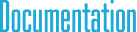#cyIPoint.h File Reference

## Detailed Description

2D, 3D, 4D, and ND integer point classes.

`#include "cyCore.h"`

## Classes

class  IPoint< TYPE, N >

class  IPoint2< TYPE >

class  IPoint3< TYPE >

class  IPoint4< TYPE >

## Typedefs

typedef IPoint2< int8_t > IPoint2b

typedef IPoint3< int8_t > IPoint3b

typedef IPoint4< int8_t > IPoint4b

typedef IPoint2< uint8_t > IPoint2ub

typedef IPoint3< uint8_t > IPoint3ub

typedef IPoint4< uint8_t > IPoint4ub

typedef IPoint2< int16_t > IPoint2s

typedef IPoint3< int16_t > IPoint3s

typedef IPoint4< int16_t > IPoint4s

typedef IPoint2< uint16_t > IPoint2us

typedef IPoint3< uint16_t > IPoint3us

typedef IPoint4< uint16_t > IPoint4us

typedef IPoint2< int32_t > IPoint2i

typedef IPoint3< int32_t > IPoint3i

typedef IPoint4< int32_t > IPoint4i

typedef IPoint2< uint32_t > IPoint2ui

typedef IPoint3< uint32_t > IPoint3ui

typedef IPoint4< uint32_t > IPoint4ui

typedef IPoint2< int64_t > IPoint2l

typedef IPoint3< int64_t > IPoint3l

typedef IPoint4< int64_t > IPoint4l

typedef IPoint2< uint64_t > IPoint2ul

typedef IPoint3< uint64_t > IPoint3ul

typedef IPoint4< uint64_t > IPoint4ul

typedef cy::IPoint2b cyIPoint2b

typedef cy::IPoint3b cyIPoint3b

typedef cy::IPoint4b cyIPoint4b

typedef cy::IPoint2ub cyIPoint2ub

typedef cy::IPoint3ub cyIPoint3ub

typedef cy::IPoint4ub cyIPoint4ub

typedef cy::IPoint2s cyIPoint2s

typedef cy::IPoint3s cyIPoint3s

typedef cy::IPoint4s cyIPoint4s

typedef cy::IPoint2us cyIPoint2us

typedef cy::IPoint3us cyIPoint3us

typedef cy::IPoint4us cyIPoint4us

typedef cy::IPoint2i cyIPoint2i

typedef cy::IPoint3i cyIPoint3i

typedef cy::IPoint4i cyIPoint4i

typedef cy::IPoint2ui cyIPoint2ui

typedef cy::IPoint3ui cyIPoint3ui

typedef cy::IPoint4ui cyIPoint4ui

typedef cy::IPoint2l cyIPoint2l

typedef cy::IPoint3l cyIPoint3l

typedef cy::IPoint4l cyIPoint4l

typedef cy::IPoint2ul cyIPoint2ul

typedef cy::IPoint3ul cyIPoint3ul

typedef cy::IPoint4ul cyIPoint4ul

## § IPoint2b

 typedef IPoint2 IPoint2b

8-bit signed integer (int8_t) 2D integer point class

## § IPoint3b

 typedef IPoint3 IPoint3b

8-bit signed integer (int8_t) 3D integer point class

## § IPoint4b

 typedef IPoint4 IPoint4b

8-bit signed integer (int8_t) 4D integer point class

## § IPoint2ub

 typedef IPoint2 IPoint2ub

8-bit unsigned integer (uint8_t) 2D integer point class

## § IPoint3ub

 typedef IPoint3 IPoint3ub

8-bit unsigned integer (uint8_t) 3D integer point class

## § IPoint4ub

 typedef IPoint4 IPoint4ub

8-bit unsigned integer (uint8_t) 4D integer point class

## § IPoint2s

 typedef IPoint2 IPoint2s

16-bit signed integer (int16_t) 2D integer point class

## § IPoint3s

 typedef IPoint3 IPoint3s

16-bit signed integer (int16_t) 3D integer point class

## § IPoint4s

 typedef IPoint4 IPoint4s

16-bit signed integer (int16_t) 4D integer point class

## § IPoint2us

 typedef IPoint2 IPoint2us

16-bit unsigned integer (uint16_t) 2D integer point class

## § IPoint3us

 typedef IPoint3 IPoint3us

16-bit unsigned integer (uint16_t) 3D integer point class

## § IPoint4us

 typedef IPoint4 IPoint4us

16-bit unsigned integer (uint16_t) 4D integer point class

## § IPoint2i

 typedef IPoint2 IPoint2i

32-bit signed integer (int32_t) 2D integer point class

## § IPoint3i

 typedef IPoint3 IPoint3i

32-bit signed integer (int32_t) 3D integer point class

## § IPoint4i

 typedef IPoint4 IPoint4i

32-bit signed integer (int32_t) 4D integer point class

## § IPoint2ui

 typedef IPoint2 IPoint2ui

32-bit unsigned integer (uint32_t) 2D integer point class

## § IPoint3ui

 typedef IPoint3 IPoint3ui

32-bit unsigned integer (uint32_t) 3D integer point class

## § IPoint4ui

 typedef IPoint4 IPoint4ui

32-bit unsigned integer (uint32_t) 4D integer point class

## § IPoint2l

 typedef IPoint2 IPoint2l

64-bit signed integer (int64_t) 2D integer point class

## § IPoint3l

 typedef IPoint3 IPoint3l

64-bit signed integer (int64_t) 3D integer point class

## § IPoint4l

 typedef IPoint4 IPoint4l

64-bit signed integer (int64_t) 4D integer point class

## § IPoint2ul

 typedef IPoint2 IPoint2ul

64-bit unsigned integer (uint64_t) 2D integer point class

## § IPoint3ul

 typedef IPoint3 IPoint3ul

64-bit unsigned integer (uint64_t) 3D integer point class

## § IPoint4ul

 typedef IPoint4 IPoint4ul

64-bit unsigned integer (uint64_t) 4D integer point class

## § cyIPoint2b

 typedef cy::IPoint2b cyIPoint2b

8-bit signed integer (int8_t) 2D integer point class

## § cyIPoint3b

 typedef cy::IPoint3b cyIPoint3b

8-bit signed integer (int8_t) 3D integer point class

## § cyIPoint4b

 typedef cy::IPoint4b cyIPoint4b

8-bit signed integer (int8_t) 4D integer point class

## § cyIPoint2ub

 typedef cy::IPoint2ub cyIPoint2ub

8-bit unsigned integer (uint8_t) 2D integer point class

## § cyIPoint3ub

 typedef cy::IPoint3ub cyIPoint3ub

8-bit unsigned integer (uint8_t) 3D integer point class

## § cyIPoint4ub

 typedef cy::IPoint4ub cyIPoint4ub

8-bit unsigned integer (uint8_t) 4D integer point class

## § cyIPoint2s

 typedef cy::IPoint2s cyIPoint2s

16-bit signed integer (int16_t) 2D integer point class

## § cyIPoint3s

 typedef cy::IPoint3s cyIPoint3s

16-bit signed integer (int16_t) 3D integer point class

## § cyIPoint4s

 typedef cy::IPoint4s cyIPoint4s

16-bit signed integer (int16_t) 4D integer point class

## § cyIPoint2us

 typedef cy::IPoint2us cyIPoint2us

16-bit unsigned integer (uint16_t) 2D integer point class

## § cyIPoint3us

 typedef cy::IPoint3us cyIPoint3us

16-bit unsigned integer (uint16_t) 3D integer point class

## § cyIPoint4us

 typedef cy::IPoint4us cyIPoint4us

16-bit unsigned integer (uint16_t) 4D integer point class

## § cyIPoint2i

 typedef cy::IPoint2i cyIPoint2i

32-bit signed integer (int32_t) 2D integer point class

## § cyIPoint3i

 typedef cy::IPoint3i cyIPoint3i

32-bit signed integer (int32_t) 3D integer point class

## § cyIPoint4i

 typedef cy::IPoint4i cyIPoint4i

32-bit signed integer (int32_t) 4D integer point class

## § cyIPoint2ui

 typedef cy::IPoint2ui cyIPoint2ui

32-bit unsigned integer (uint32_t) 2D integer point class

## § cyIPoint3ui

 typedef cy::IPoint3ui cyIPoint3ui

32-bit unsigned integer (uint32_t) 3D integer point class

## § cyIPoint4ui

 typedef cy::IPoint4ui cyIPoint4ui

32-bit unsigned integer (uint32_t) 4D integer point class

## § cyIPoint2l

 typedef cy::IPoint2l cyIPoint2l

64-bit signed integer (int64_t) 2D integer point class

## § cyIPoint3l

 typedef cy::IPoint3l cyIPoint3l

64-bit signed integer (int64_t) 3D integer point class

## § cyIPoint4l

 typedef cy::IPoint4l cyIPoint4l

64-bit signed integer (int64_t) 4D integer point class

## § cyIPoint2ul

 typedef cy::IPoint2ul cyIPoint2ul

64-bit unsigned integer (uint64_t) 2D integer point class

## § cyIPoint3ul

 typedef cy::IPoint3ul cyIPoint3ul

64-bit unsigned integer (uint64_t) 3D integer point class

## § cyIPoint4ul

 typedef cy::IPoint4ul cyIPoint4ul

64-bit unsigned integer (uint64_t) 4D integer point class